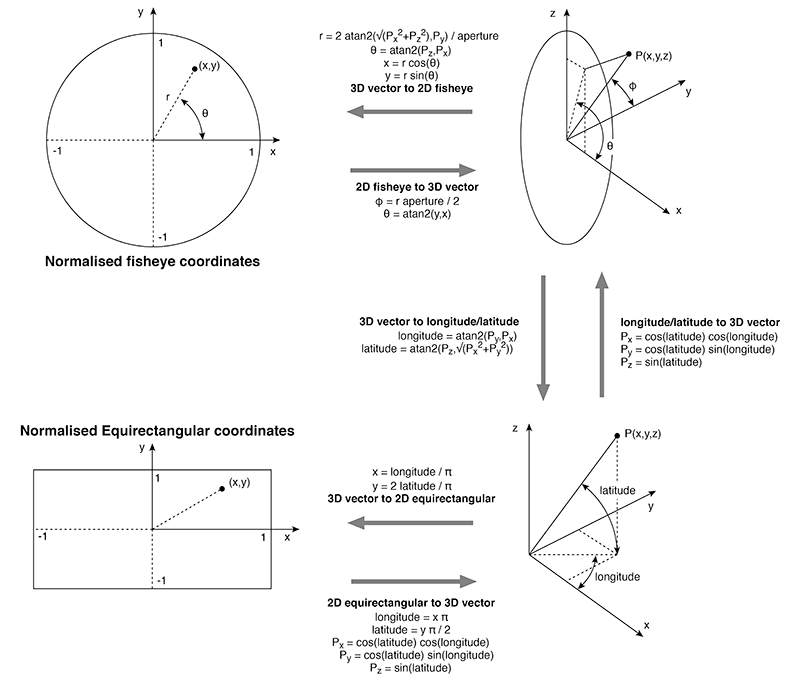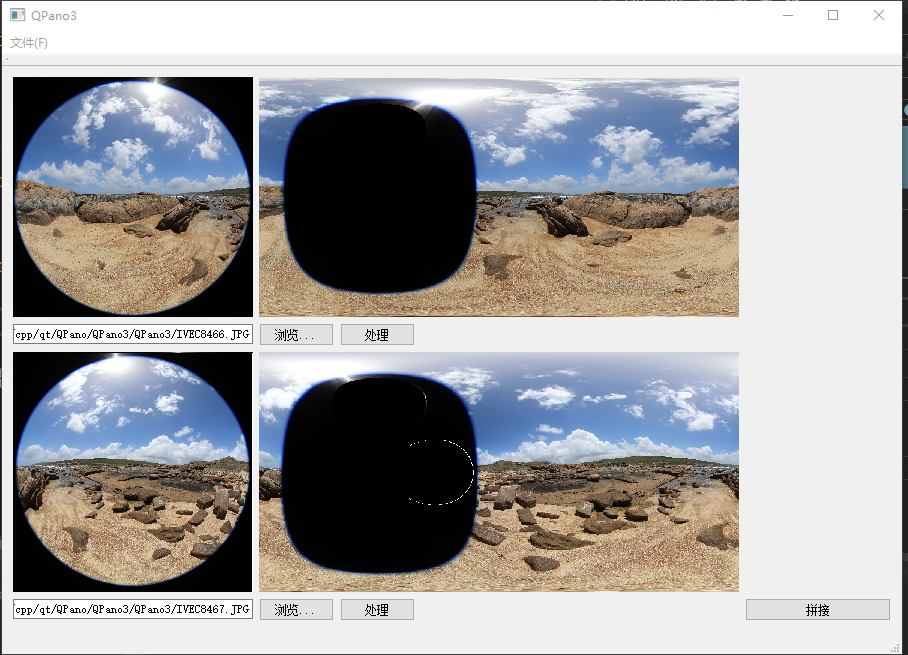# 理论部分## 鱼眼标准坐标计算

OpenCv读取一张鱼眼图片，其图片数据可以想象成发布在一个2D的笛卡尔坐标中的像素点的集合：x \in [ 0,cols-1] y \in [ 0,cols-1]将输入的鱼眼图片坐标换算成标准鱼眼坐标：normalCoord.x = (float)((float)x * 2 / cols – 1 ) normalCoord.y = (float)((float)y * 2 / rows – 1 )

## 标准坐标系与球坐标的转换

p :点P与原点O连线的径向距离，下面即用OP表示；
\theta :OP与Z轴之间的夹角；
\phi :OP在XOY平面的投影与正X轴的夹角；**

x = p * sin\theta * cos\phi y = p * sin\theta * sin \phi z = p * cos\theta

# 代码实现

FishEye& FishEye::ImgExpand2()
{
if (mImg.empty()) {
return *this;
}
printf("Current x is (%d ,%d)\n", mImg.cols, mImg.rows);

Point2i circleCoord;
circleCoord.x = mImg.cols / 2 + 2;
circleCoord.y = mImg.rows / 2 + 5;
int R = mImg.cols / 2;

for (int i = 0; i < mDesImg.cols; i++) {
for (int j = 0; j < mDesImg.rows; j++) {

Point2f  pointDst = this->Shpere2Fish(i, j);
int cols = (int)((pointDst.x + 1) * mImg.cols / 2);
int rows = (int)((pointDst.y + 1) * mImg.rows / 2);
printf("Current %d %d is (%d, %d) ", i, j, cols, rows);
if (rows < mImg.rows && cols < mImg.cols
&& rows >= 0 && cols >= 0) {
Vec3b color_value = mImg.at<Vec3b>(rows, cols);
mDesImg.at<Vec3b>(j, i) = color_value;
std::cout << color_value << std::endl;
}
}
}
return *this;
}

cv::Point2f FishEye::Shpere2Fish(int x, int y)
{
//归一化
Point2f normalCoord;
normalCoord.x = (float)(x * 2.0f / mFishMapImg.cols - 1);
normalCoord.y = (float)(y * 2.0f / mFishMapImg.rows - 1);

float longitude = (float)(normalCoord.x * PI);
float latitude = (float)(normalCoord.y * PI/2);

Point3f coordP;
coordP.x = cos(latitude)*cos(longitude);
coordP.y = cos(latitude)*sin(longitude);
coordP.z = sin(latitude);
float r = 2 * atan2(sqrt(coordP.x*coordP.x + coordP.z*coordP.z), coordP.y) / MFOV;
float theta = atan2(coordP.z, coordP.x);

Point2f fishCoord;
fishCoord.x = r * cos(theta);
fishCoord.y = r * sin(theta);

return fishCoord;
}


## 测试效果如下图34 views